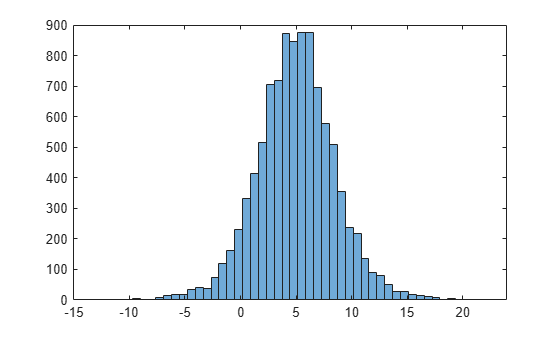Documentation

# LoglogisticDistribution

Loglogistic probability distribution object

## Description

A `LoglogisticDistribution` object consists of parameters, a model description, and sample data for a loglogistic probability distribution.

The loglogistic distribution is closely related to the logistic distribution. If x is distributed loglogistically with parameters μ and σ, then log(x) is distributed logistically with mean and standard deviation. This distribution is often used in survival analysis to model events that experience an initial rate increase, followed by a rate decrease.

The loglogistic distribution uses the following parameters.

ParameterDescriptionSupport
`mu`Mean of logarithmic values$\mu >0$
`sigma`Scale parameter of logarithmic values$\sigma >0$

## Creation

There are several ways to create a `LoglogisticDistribution` probability distribution object.

## Properties

expand all

### Distribution Parameters

Mean of logarithmic values for the loglogistic distribution, specified as a positive scalar value.

Data Types: `single` | `double`

Scale parameter of logarithmic values for the loglogistic distribution, specified as a positive scalar value.

Data Types: `single` | `double`

### Distribution Characteristics

This property is read-only.

Logical flag for truncated distribution, specified as a logical value. If `IsTruncated` equals `0`, the distribution is not truncated. If `IsTruncated` equals `1`, the distribution is truncated.

Data Types: `logical`

This property is read-only.

Number of parameters for the probability distribution, specified as a positive integer value.

Data Types: `double`

This property is read-only.

Covariance matrix of the parameter estimates, specified as a p-by-p matrix, where p is the number of parameters in the distribution. The (`i`,`j`) element is the covariance between the estimates of the `i`th parameter and the `j`th parameter. The (`i`,`i`) element is the estimated variance of the `i`th parameter. If parameter `i` is fixed rather than estimated by fitting the distribution to data, then the (`i`,`i`) elements of the covariance matrix are 0.

Data Types: `double`

This property is read-only.

Logical flag for fixed parameters, specified as an array of logical values. If `0`, the corresponding parameter in the `ParameterNames` array is not fixed. If `1`, the corresponding parameter in the `ParameterNames` array is fixed.

Data Types: `logical`

This property is read-only.

Distribution parameter values, specified as a vector.

Data Types: `single` | `double`

This property is read-only.

Truncation interval for the probability distribution, specified as a vector containing the lower and upper truncation boundaries.

Data Types: `single` | `double`

### Other Object Properties

This property is read-only.

Probability distribution name, specified as a character vector.

Data Types: `char`

This property is read-only.

Data used for distribution fitting, specified as a structure containing the following:

• `data`: Data vector used for distribution fitting.

• `cens`: Censoring vector, or empty if none.

• `freq`: Frequency vector, or empty if none.

Data Types: `struct`

This property is read-only.

Distribution parameter descriptions, specified as a cell array of character vectors. Each cell contains a short description of one distribution parameter.

Data Types: `char`

This property is read-only.

Distribution parameter names, specified as a cell array of character vectors.

Data Types: `char`

## Object Functions

 `cdf` Cumulative distribution function `icdf` Inverse cumulative distribution function `iqr` Interquartile range `mean` Mean of probability distribution `median` Median of probability distribution `negloglik` Negative loglikelihood of probability distribution `paramci` Confidence intervals for probability distribution parameters `pdf` Probability density function `proflik` Profile likelihood function for probability distribution `random` Random numbers `std` Standard deviation of probability distribution `truncate` Truncate probability distribution object `var` Variance of probability distribution

## Examples

collapse all

Create a loglogistic distribution object using the default parameter values.

`pd = makedist('Loglogistic')`
```pd = LoglogisticDistribution Log-Logistic distribution mu = 0 sigma = 1 ```

Create a loglogistic distribution object by specifying the parameter values.

`pd = makedist('Loglogistic','mu',5,'sigma',2)`
```pd = LoglogisticDistribution Log-Logistic distribution mu = 5 sigma = 2 ```

Generate random numbers from the loglogistic distribution and compute their log values.

```rng(19) % for reproducibility x = random(pd,10000,1); logx = log(x);```

Compute the mean of the log values.

`m = mean(logx)`
```m = 4.9828 ```

The mean of the log of `x` is equal to the `mu` parameter of `x`, since `x` has a loglogistic distribution.

Plot `logx`.

`histogram(logx,50)`The plot shows that the log values of `x` have a logistic distribution.